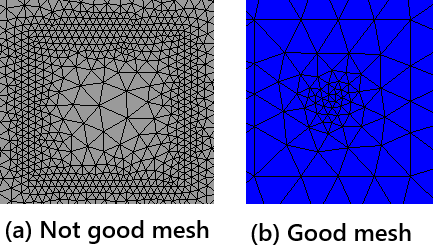# Visualizing magnetic vector potential A

Hello,

I have a question about the magnetic vector potential A not being displayed correctly.

The A is needed to calculate magnetic flux through a region of space enclosed by a coil.
I have tried to display A in the following two tutorials using ver. 6.2.2304.

An attempt to do this can be easily accomplished by modifying the Draw command as follows.

``````Draw (u, mesh, "A-field")
``````

The direction of A must be equal to the direction of I or curl M, correct?
In “2.4 Maxwell’s Equations”, the direction is displayed correctly.
In “Symbolic definition of forms : magnetic field”, the direction is not displayed correctly.

It seems that A may not be displayed correctly when “f” is given in terms of current density.

I could be wrong.
Because B is displayed correctly in the two tutorials.
I must be grateful if anyone would be send comments.

Thank you, Daigo

Hello,

To obtain the correct A, I found that it was important that the value of order in the following command be greater than 1.

``````with TaskManager():
mesh = Mesh(ngmesh)
############################################################################
mesh.Curve(order=5) # Important command for getting A
############################################################################
``````

However, I don’t understand why this command has so much less impact on B than it does on A.
Even if the order is 1, good B is obtained.

I hope this helps you.

Best, Daigo

What you see here is gradient fields. since curl(grad(u)) = 0 they are unseen by B.
If your right hand side is not numerically div free these gradient fields are large due to the regularization mass term on the left hand side. By curving the geometry the right hand side becomes numerically div free.
Another option would be to use a mixed formulation where you actively impose u * grad(v) = 0 and do not need a regularization.

Best
Christopher

Dear Christopher,

Your comment helped me a lot.
I understand that implementing `u * grad(v) = 0` requires some ingenuity.
Because `a += SymbolicBFI(u*grad(v))` will raise the error `NgException: Dimensions don’t match’. I need to study electromagnetics further.

1. I am interested in the mathematical meaning of the coefficient in front of “nu”.
The following tutorials almost cancel out “nu”, where nu=7.96e5.
``````a += nu*curl(u)*curl(v)*dx + 1e-6*nu*u*v*dx
``````
``````a += 1/(mu0*mur)*curl(u)*curl(v)*dx + 1e-8/(mu0*mur)*u*v*dx
``````
``````a = BilinearForm(1/mu*curl(u)*curl(v)*dx+1e-6/mu*u*v*dx)
``````

However, the following command in my application gives me good results for the A.

``````a += nu*curl(u)*curl(v)*dx + nu*u*v*dx
``````
1. The mesh of a coil area to be contour-integrated must be fine enough.
This is because the mesh on the line to be contour integrated must be fine enough to represent A.• Not good mesh. Because mesh around the inside of the cross-section of the coil isn’t fine.
``````def makeCoil_NotGood(radius:float, width:float, height:float, p=(0,0,0)):
p_out = (0+p, -height/2+p, 0+p)
coil.maxh = dr
coil.mat('coil')
coil.faces.col = (1,1,0)
return coil
``````
• Good mesh. Because mesh around the inside of the cross-section of the coil is fine.
``````def makeCoil(radius:float, width:float, height:float, p=(0,0,0)):
p_out = (0+p, -height/2+p, 0+p)
#######################################
# inside of cross section of the coil
p_in = (0+p, -dr/2+p, 0+p)